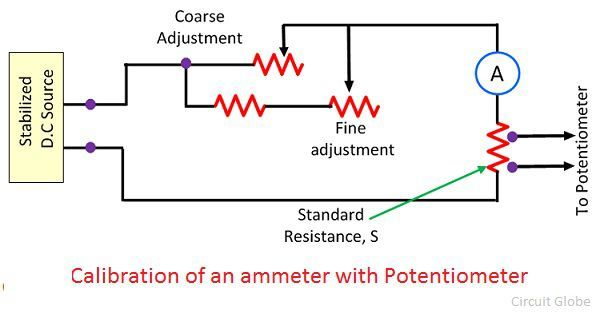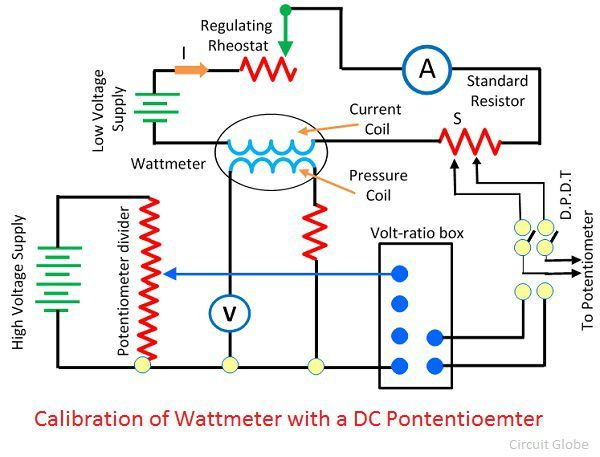# Calibration of Voltmeter, Ammeter & Wattmeter using Potentiometer

The calibration is the process of checking the accuracy of the result by comparing it with the standard value. In other words, calibration checks the correctness of the instrument by comparing it with the reference standard. It helps us in determining the error occur in the reading and adjusts the voltages for getting the ideal reading.

### Calibration of Voltmeter

The circuit for the calibration of the voltmeter is shown in the figure below.The circuit requires two rheostats, one for controlling the voltage and another for adjustment. The voltage ratio box is used to step-down the voltage to a suitable value. The accurate value of the voltmeter is determined by measuring the value of the voltage to the maximum possible range of the potentiometer.

The potentiometer measures the maximum possible value of voltages. The negative and positive error occurs in the readings of the voltmeter if the readings of the potentiometer and the voltmeter are not equal.

### Calibration of Ammeter

The figure below shows the circuit for the calibration of the ammeter.The standard resistance is connected in series with the ammeter which is to be calibrated. The potentiometer is used for measuring the voltage across the standard resistor. The below mention formula determines the current through the standard resistance.Where,
Vs – voltage across the standard resistor as indicated by the potentiometer.
S – resistance of standard resistor

This method of calibration of the ammeter is very accurate because in this method the value of standard resistance and the voltage across the potentiometer is exactly known by the instrument.

### Calibrating of Wattmeter

The figure below shows the circuit used for calibrating the Wattmeter.The standard resistance is connected in series with the Wattmeter which is to be calibrated. The low voltage supply is given to the current coil of the Wattmeter. The rheostat is connected in series with the coil for adjusting the value of current.

The potential circuit is supplied from the supply. The volt-ratio box is used to step-down the voltage so that the potentiometer can easily read the voltage. The actual value of the actual value of voltage and current is measured by using a double pole double throw switch. The accurate value VI and the value of Wattmeter are compared.